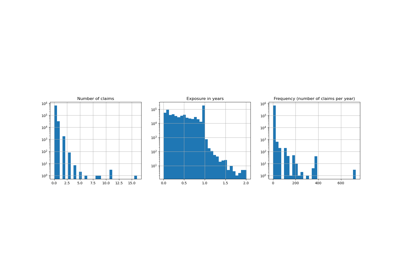# sklearn.dummy.DummyRegressor¶

class sklearn.dummy.DummyRegressor(*, strategy='mean', constant=None, quantile=None)[source]

DummyRegressor is a regressor that makes predictions using simple rules.

This regressor is useful as a simple baseline to compare with other (real) regressors. Do not use it for real problems.

Read more in the User Guide.

New in version 0.13.

Parameters
strategystr

Strategy to use to generate predictions.

• “mean”: always predicts the mean of the training set

• “median”: always predicts the median of the training set

• “quantile”: always predicts a specified quantile of the training set, provided with the quantile parameter.

• “constant”: always predicts a constant value that is provided by the user.

constantint or float or array-like of shape (n_outputs,)

The explicit constant as predicted by the “constant” strategy. This parameter is useful only for the “constant” strategy.

quantilefloat in [0.0, 1.0]

The quantile to predict using the “quantile” strategy. A quantile of 0.5 corresponds to the median, while 0.0 to the minimum and 1.0 to the maximum.

Attributes
constant_array, shape (1, n_outputs)

Mean or median or quantile of the training targets or constant value given by the user.

n_outputs_int,

Number of outputs.

Examples

>>> import numpy as np
>>> from sklearn.dummy import DummyRegressor
>>> X = np.array([1.0, 2.0, 3.0, 4.0])
>>> y = np.array([2.0, 3.0, 5.0, 10.0])
>>> dummy_regr = DummyRegressor(strategy="mean")
>>> dummy_regr.fit(X, y)
DummyRegressor()
>>> dummy_regr.predict(X)
array([5., 5., 5., 5.])
>>> dummy_regr.score(X, y)
0.0


Methods

 fit(X, y[, sample_weight]) Fit the random regressor. get_params([deep]) Get parameters for this estimator. predict(X[, return_std]) Perform classification on test vectors X. score(X, y[, sample_weight]) Returns the coefficient of determination R^2 of the prediction. set_params(**params) Set the parameters of this estimator.
__init__(*, strategy='mean', constant=None, quantile=None)[source]

Initialize self. See help(type(self)) for accurate signature.

fit(X, y, sample_weight=None)[source]

Fit the random regressor.

Parameters
X{array-like, object with finite length or shape}

Training data, requires length = n_samples

yarray-like of shape (n_samples,) or (n_samples, n_outputs)

Target values.

sample_weightarray-like of shape (n_samples,), default=None

Sample weights.

Returns
selfobject
get_params(deep=True)[source]

Get parameters for this estimator.

Parameters
deepbool, default=True

If True, will return the parameters for this estimator and contained subobjects that are estimators.

Returns
paramsmapping of string to any

Parameter names mapped to their values.

predict(X, return_std=False)[source]

Perform classification on test vectors X.

Parameters
X{array-like, object with finite length or shape}

Training data, requires length = n_samples

return_stdboolean, optional

Whether to return the standard deviation of posterior prediction. All zeros in this case.

New in version 0.20.

Returns
yarray-like of shape (n_samples,) or (n_samples, n_outputs)

Predicted target values for X.

y_stdarray-like of shape (n_samples,) or (n_samples, n_outputs)

Standard deviation of predictive distribution of query points.

score(X, y, sample_weight=None)[source]

Returns the coefficient of determination R^2 of the prediction.

The coefficient R^2 is defined as (1 - u/v), where u is the residual sum of squares ((y_true - y_pred) ** 2).sum() and v is the total sum of squares ((y_true - y_true.mean()) ** 2).sum(). The best possible score is 1.0 and it can be negative (because the model can be arbitrarily worse). A constant model that always predicts the expected value of y, disregarding the input features, would get a R^2 score of 0.0.

Parameters
X{array-like, None}

Test samples with shape = (n_samples, n_features) or None. For some estimators this may be a precomputed kernel matrix instead, shape = (n_samples, n_samples_fitted], where n_samples_fitted is the number of samples used in the fitting for the estimator. Passing None as test samples gives the same result as passing real test samples, since DummyRegressor operates independently of the sampled observations.

yarray-like of shape (n_samples,) or (n_samples, n_outputs)

True values for X.

sample_weightarray-like of shape (n_samples,), default=None

Sample weights.

Returns
scorefloat

R^2 of self.predict(X) wrt. y.

set_params(**params)[source]

Set the parameters of this estimator.

The method works on simple estimators as well as on nested objects (such as pipelines). The latter have parameters of the form <component>__<parameter> so that it’s possible to update each component of a nested object.

Parameters
**paramsdict

Estimator parameters.

Returns
selfobject

Estimator instance.

## Examples using sklearn.dummy.DummyRegressor¶